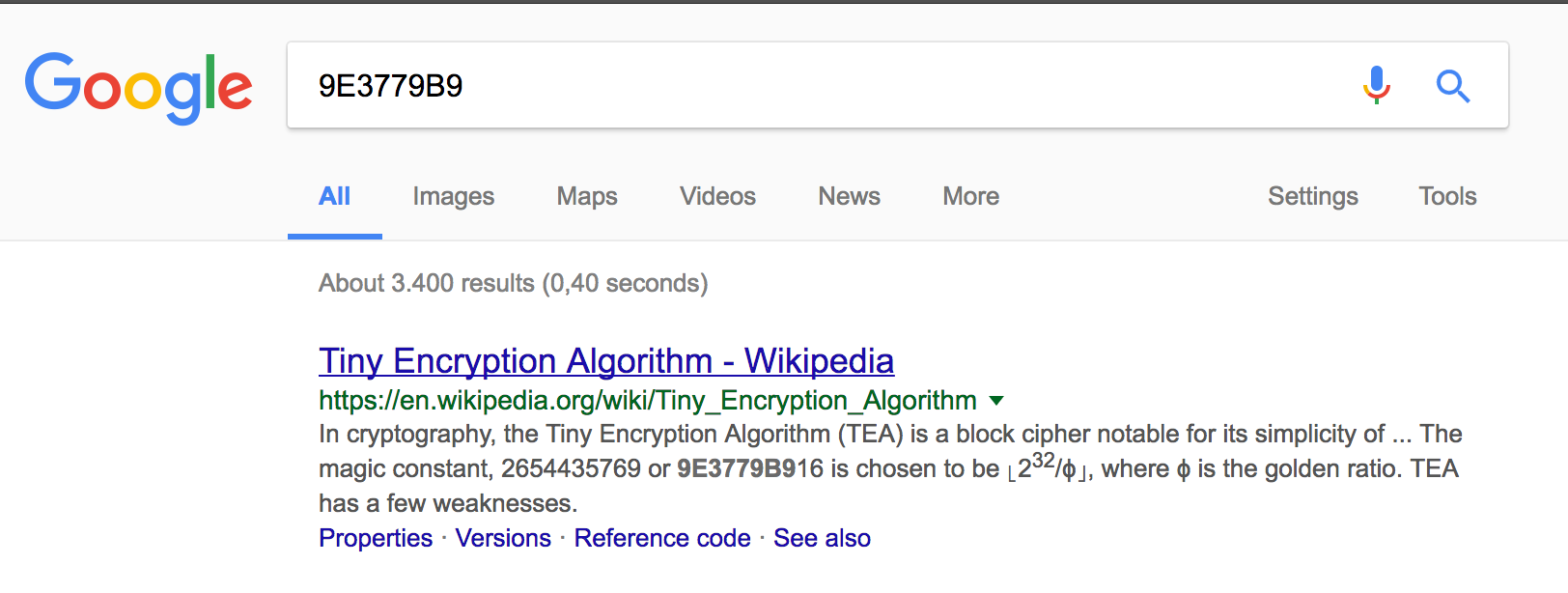# CSCBE Challenge Write-up – Sufbo

The Sufbo challenge was tackled during the Cyber Security Challenge qualifiers and proved to be very difficult to solve. This write-up gives you a possible way of solving it!

Credits

All challenges of the Cyber Security Challenge are created by security professionals from many different organisations. The Sufbo challenge in particular was created by Adriaan Dens, one of our distinguished challenge contributors, from Proximus. Adriaan & Proximus have contributed multiple challenges over the years and they tend to be pretty hard to solve ;).

The challenge

And you thought Assembly was hard to read? Try this!

The solution

The challenge consists out of a heavily obfuscated piece of perl code. We can start by cleaning up the code which improves the readability by a small bit:

```print"Flag: ";
chomp(\$_=&amp;lt;&amp;gt;);
\$[=0;
die if y///c!=32;
chomp(@_=&amp;lt;DATA&amp;gt;);
\$/=join'',map{chr(ord\$_^\$=)}split//,pack"H*",shift(@_).shift(@_);

while(\$,=substr\$_,8*\$-,8) {
(\$@,\$*,\$#,\$x,\$y,\$z,\$!,\$.,\$,) = (unpack("N*",\$/.\$,),0,2**31*(sqrt(5)-1),(1&amp;lt;&amp;lt;32)-1);
map {
\$!+=\$.;
\$!&amp;amp;=\$,;
\$y+=(((((\$z&amp;lt;&amp;lt;4)&amp;amp;\$,)+\$@)&amp;amp;\$,)^((\$z+\$!)&amp;amp;\$,)^(((\$z&amp;gt;&amp;gt;5)+\$*)&amp;amp;\$,));
\$y&amp;amp;=\$,;
\$z+=(((((\$y&amp;lt;&amp;lt;4)&amp;amp;\$,)+\$#)&amp;amp;\$,)^((\$y+\$!)&amp;amp;\$,)^(((\$y&amp;gt;&amp;gt;5)+\$x)&amp;amp;\$,));
\$z&amp;amp;=\$,;
\$"=pack("N*",\$y,\$z);
\$/=\$"x2
}0..31;
die if\$"ne pack"H*",\$_[\$-];
\$-++;
}
print "OK\n"

__DATA__
6c594e50630d4f63
7d515d4655525b1d
7872575285c742da
15c670798094a00b
54f08c6b937ed1f2
6810afed7372cd76
```

This code might still not mean a lot to the average non-perl-speaking-person. Let’s take a look at the same code, but with some inline comments:

```print"Flag: "; # Prints "Flag: " to STDIN
chomp(\$_=&amp;lt;&amp;gt;); # Reads in the input into the variable \$_
\$[=0; # Changes the starting index of an array to 0 (It's a useless command actually)
die if y///c!=32; # y///c is a Perl golfing idiom that is similar to length(\$_), so the length of your input has to be a string of length 32.
chomp(@_=&amp;lt;DATA&amp;gt;); # Store the data below (under __DATA__) in the array @_
\$/=join'',map{chr(ord\$_^\$=)}split//,pack"H*",shift(@_).shift(@_); # Shift the first two elements of @_, "unhexify" the strings, split them per character, XOR with \$= (default value is 60), and join the characters back in the variable \$/.
#
while(\$,=substr\$_,8*\$-,8) { # While there are 8 characters left in the input do:
(\$@,\$*,\$#,\$x,\$y,\$z,\$!,\$.,\$,) = (unpack("N*",\$/.\$,),0,2**31*(sqrt(5)-1),(1&amp;lt;&amp;lt;32)-1); # Convert the variable \$/ (unknown) and \$, (our input) to unsigned numbers, assign 0 to \$!, assign 2**31*(sqrt(5)-1) to \$/ and assign (1&amp;lt;&amp;lt;32)-1 to \$,.
map { # Use map to loop 32 times (see below)
\$!+=\$.; # Add \$. to \$!
\$!&amp;amp;=\$,; # Bitwise AND \$! with \$,
\$y+=(((((\$z&amp;lt;&amp;lt;4)&amp;amp;\$,)+\$@)&amp;amp;\$,)^((\$z+\$!)&amp;amp;\$,)^(((\$z&amp;gt;&amp;gt;5)+\$*)&amp;amp;\$,)); # Some bitwise operations added to \$y
\$y&amp;amp;=\$,; # Bitwise AND \$y with \$,
\$z+=(((((\$y&amp;lt;&amp;lt;4)&amp;amp;\$,)+\$#)&amp;amp;\$,)^((\$y+\$!)&amp;amp;\$,)^(((\$y&amp;gt;&amp;gt;5)+\$x)&amp;amp;\$,)); # Some bitwise operations added to \$z
\$z&amp;amp;=\$,; # Bitwise AND \$z with \$,
\$"=pack("N*",\$y,\$z); # Convert the unsigned numbers back to string representation
\$/=\$"x2 # Set \$/ to two times \$"
}0..31; # Use map to loop 32 times
die if\$"ne pack"H*",\$_[\$-]; # Die if \$" is not equal to the "unhexified" element (\$- contains the index) in @_
\$-++; # Increase the variable \$-
} #
print "OK\n" # Printed if you have the key
#
__DATA__ # Starting the DATA block (kinda like a here document)
6c594e50630d4f63 # This part was used for \$/ in line 6.
7d515d4655525b1d # This part was used for \$/ in line 6.
7872575285c742da # This part was used to compare with the input on line 20
15c670798094a00b # This part was used to compare with the input on line 20
54f08c6b937ed1f2 # This part was used to compare with the input on line 20
6810afed7372cd76 # This part was used to compare with the input on line 20
```

So now we more or less know what each line does but we still miss context on a higher level (what it is doing). As always in reverse engineering, you try to find some “known parts” which allow you to understand the code a lot faster. These parts are usually strings, metadata, fixed numbers or familiar code blocks.

In our case, we have 2 fixed numbers:$2**31*(sqrt(5)-1)$ and$(1<<32)-1$. In this representation they don’t mean much but if we convert them to hex numbers we get 0x9e3779b9 and 0xffffffff respectively.Hmm, interesting! Seems like we’ve got a Perl implementation of Tiny Encryption Algorithm (TEA) on our hands here!

More specifically, the while loop block in the code is the actual TEA implementation, which decrypts the second half of the __DATA__ section using the first half as the key.

Retrieving the key can be done using the following perl one-liner:

```perl -E 'say join"",map{chr(ord\$_^\$=)}split//,pack"H*","6c594e50630d4f637d515d4655525b1d"
```

Which yields us “Perl_1s_Amazing!” as the key.

So now we have they key and the data to be decrypted. Let’s be lazy and copy the reference code listed on the wikipedia page we found earlier.

```#include&amp;lt;stdint.h&amp;gt;
#include&amp;lt;stdio.h&amp;gt;

void decrypt (uint32_t* v, uint32_t* k) {
uint32_t v0=v, v1=v, sum=0xC6EF3720, i; /* set up */
uint32_t delta=0x9e3779b9; /* a key schedule constant */
uint32_t k0=k, k1=k, k2=k, k3=k; /* cache key */
for (i=0; i&amp;lt;32; i++) { /* basic cycle start */
v1 -= ((v0&amp;lt;&amp;lt;4) + k2) ^ (v0 + sum) ^ ((v0&amp;gt;&amp;gt;5) + k3);
v0 -= ((v1&amp;lt;&amp;lt;4) + k0) ^ (v1 + sum) ^ ((v1&amp;gt;&amp;gt;5) + k1);
sum -= delta;
} /* end cycle */
v=v0; v=v1;
printf("%x%x", v0, v1);
}

void main() {
/* Our cipher chunks, found in the __DATA__ block of the Perl code */
uint32_t c0[] = { 0x78725752, 0x85c742da };
uint32_t c1[] = { 0x15c67079, 0x8094a00b };
uint32_t c2[] = { 0x54f08c6b, 0x937ed1f2 };
uint32_t c3[] = { 0x6810afed, 0x7372cd76 };

/* The used keys for encrypting */
uint32_t k[] = { 0x5065726c, 0x5f31735f, 0x416d617a, 0x696e6721 }; /* Original key: Perl_1s_Amazing! */
uint32_t k0[] = { 0x78725752, 0x85c742da, 0x78725752, 0x85c742da }; /* c0 . c0 */
uint32_t k1[] = { 0x15c67079, 0x8094a00b, 0x15c67079, 0x8094a00b }; /* c1 . c1 */
uint32_t k2[] = { 0x54f08c6b, 0x937ed1f2, 0x54f08c6b, 0x937ed1f2 }; /* c2 . c2 */

/* Decrypting the chunks */
decrypt(c0,k);
decrypt(c1,k0);
decrypt(c2,k1);
decrypt(c3,k2);
}
```
```\$ gcc --std=c99 solution.c
\$ ./a.out
43534342457b5065726c31735772317465306e6365526534646e33766552727d
\$ ./a.out | perl -nle 'print pack("H*", \$_)'
&amp;gt;&amp;gt;&amp;gt; CSCBE{Perl1sWr1te0nceRe4dn3veRr}
```

There we go! CSCBE{Perl1sWr1te0nceRe4dn3veRr} was the flag.